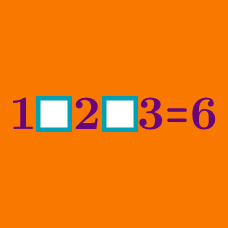Logic

# Operator Search: Level 4 Challenges

$1 \div 2\div 3 \div 4 \div 5 \div 6 \div 7$

Add one pair of parentheses to the expression above such that resultant number is an integer and is minimized. Submit your answer as this minimum number.

For instance, we could add the parentheses like $$1 \div(2\div 3 \div 4 \div 5 \div 6 \div 7) = 1260$$, the resultant number of which is an integer but is not necessarily minimized.

$1 \div 2\div 3 \div 4\div \cdots \div 12 \div 13$

Add any number of parentheses to the expression above such that the resultant number is an integer and is minimized. Submit your answer as this minimum number.

For instance, we could add 1 pair of parentheses like $$1 \div(2\div 3 \div \cdots \div13) = \dfrac{13!}4$$, the resultant number of which is an integer but is not necessarily minimized.

$\large{\begin{array}{lllllllll}&&&&&1&9&7&\square\\\times&&&&&1&0&\square&9\\\hline &&&&1&\square&8&1&1\\&&&&\square&0&0&\square&\\&&&0&\square&\square&0&&&\\&&\square&9&\square&9&&&\\ \hline &\ &1&9&\square&6&8&\square&1\\\hline\end{array}}$

Above shows an incomplete long multiplication. What is the maximum number of digits I can still remove to allow only one unique solution?

Details and Assumptions:

• For example, I can remove one of the number "1" in the very last column and I'm still able to find the unique solution. So I can remove at least more digit to allow one unique solution.

$\large 1 \, \square \, 2 \, \square \, 3 \, \square \, 4 \, \square \, 5 \, \square \, 6 \, \square \, 7 \, \square \, 8 \, \square \, 9 \, \square \, 10 = 11$

There are $$2^9 = 512$$ ways in which we can fill the squares with $$+ , -$$.

How many ways would make the equation true?

Note: You are not allowed to use parenthesis.

$\LARGE 1 \ \ \ \ \ 2 \ \ \ \ \ 3 \ \ \ \ \ 4 \ \ = \ \ 1000$

Without touching the right side of the equation, find a way to make the equation above true. You are only allowed to use mathematical operators like addition, subtraction, multiplication, division and parenthesis, percentage, and factorials only.

With your answer, define $$N$$ as the least amount of mathematical operators used. Find $$N$$.

Details and Assumptions:

• As an explicit example, if the equation $$1 \div (2 + 3)^{4!}= 1000$$ is true, then you have used 4 operators: one division, one addition, one parenthesis, one factorial. In this case, $$N=4$$.

• You can combine the digits as well. i.e.: $$12 +34$$.

• You must keep the digits in order (from left to right). $$1+2+3\times4$$ is valid while $$1+2-4\div3$$ is not valid.

• BODMAS applied.

• You need a number before the percentage sign. For example. $$1234 + \%$$ is invalid while $$123 + 4\%$$ is valid.

• Exponentiation is allowed.

×

Problem Loading...

Note Loading...

Set Loading...